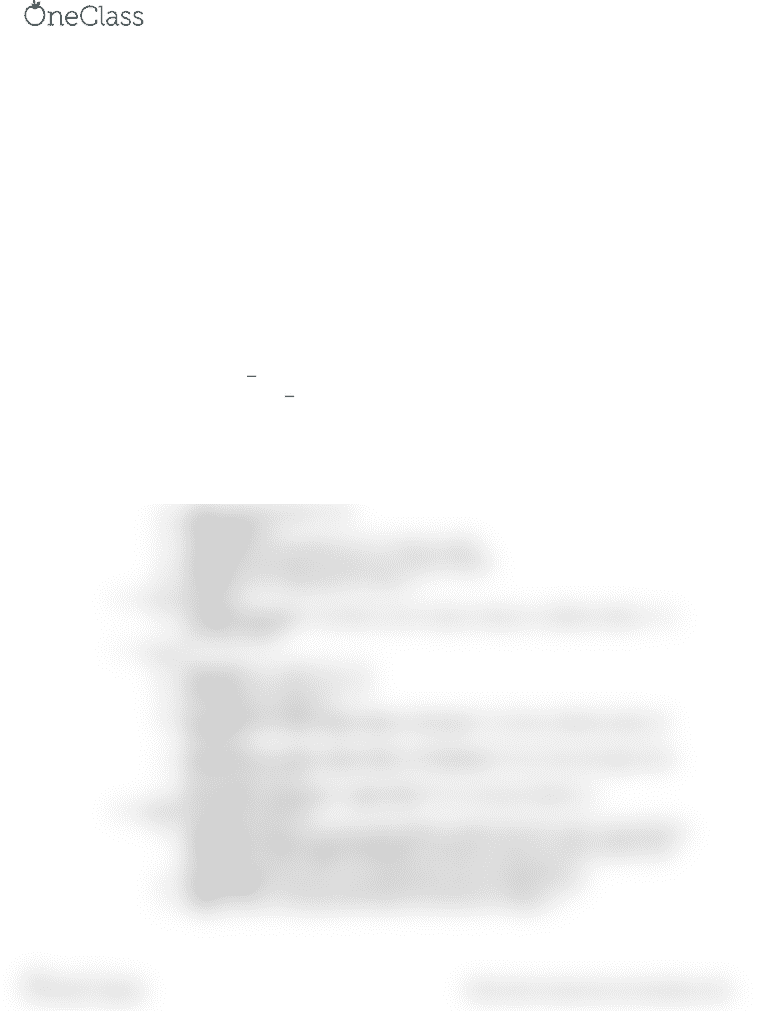# CIVE121 Study Guide - Quiz Guide: Binary Logarithm, Dot Product, Matlab

41 views2 pages1. Trigonometric functions
a. sin, cos, tan, sec, csc, cot standard in radians,
b. add d to end for degrees
c. add a to front for inverse
2. Rounding functions
a. floor (down)
b. ceil (up)
c. fix (zero)
d. round (normal rounding)
3. Remainder function
a. rem (a,b) finds remainder of a/b
4. Exponential functions
a. exp(a)=e^a
b. log(a)=ln(a)
c. log10(a)=
d. log2(a)=
e. sqrt(a)=
f. nthroot(a,b)=
g. a^b=
5. Other common functions
a. abs(a)=
b. factor(a)=prime factors of a in a row vector
c. primes(a)=prime numbers a in a row vector
d. rat(a)=rational approx of a
e. factorial(a)=a!
f. cross(a,b)=cross product of 1x3 vectors a and b
g. dot(a,b)=dot product of same size vectors a and b
h. norm(a) gives magnitude of vector a
6. User interaction
a. a=input(‘message’, ‘s’); allows user to input a string to a, default without ‘s’ is
entering double
7. Vectors
a. [a,b,c,d]=row vector a, b, c, d
b. [a;b;c;d]=col vector a, b, c, d
c. a’=transpose of matrix a
d. linspace(lower limit, upper limit, # of terms) *# of terms=default number of
100 terms
e. logspace(lower limit, upper limit, # of elements) *lower limit and upper limit
are to powers of 10
f. lower limit : increment : upper limit *if no increment default is 1
8. Random number generation
a. rand(a,b)=makes axb vector with random number between 0 and 1 non inclusive,
randi([lower limit, upper limit],a,b) *generates inclusive whole numbers and
populates an array with a rows and b cols *if no a,b generates 1 #
b. rng(‘shuffle’) create new rng algorithm based on computer time
c. rng(a) create a rng algorithm based on the positive integer a
find more resources at oneclass.com
find more resources at oneclass.com
Unlock document

This preview shows half of the first page of the document.
Unlock all 2 pages and 3 million more documents.